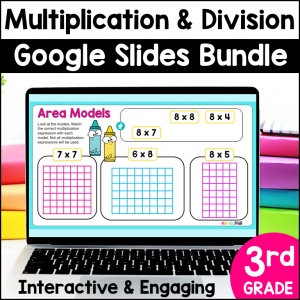Save 10% on your first order with code EXTRA10

# Division Word Problems – Taking Out Equal Groups – Measurement Division

\$2.50\$29.99\$13.49\$151.25
SKU: 8541907 Category:

## Description

Students will love these fun & engaging slides to understand division by taking out equal sets. These Google Slides include problems using equal groups division situations, where the number in each group is known and students are solving to find the number of groups.

Video instructions for students are embedded in the activity, making it easy for you to assign as a no prep math station, for seatwork or homework.

This resource can be easily assigned in Google Classroom or any other platform compatible with Google Slides. Homeschool parents can use this resource by simply opening it in Google Slides. All you need to access Google Slides is a free Gmail account.

What’s Included in this resource?

• Video Instructions (Student devices must be able to access You-Tube)
• 10 student practice slides (10 problems)
• Key

Topics Include:

• Modeling taking out equal sets division situations, also known as measurement division
• Solving division problems where the number of groups is not known
• Identifying the total, number of groups and number in each group in division equations

Ideas on how to use this resource:

• Use it as a whole class teaching tool by projecting the slides and using the movable, interactive pieces to teach your lesson
• Use this resource as a no prep math station
• Use it as an early finisher activity
• Use this resource for small group reteach or tutoring
• Assign this resource as a lesson for student practice as independent work
• Share the digital key for parents and students to check work at home

Standards Alignment:

TEKS

3.4H Determine the number of objects in each group when a set of objects is partitioned into equal shares or a set of objects is shared equally.

Common Core

3.OA.A.2 Interpret whole-number quotients of whole numbers, e.g., interpret 56 ÷ 8 as the number of objects in each share when 56 objects are partitioned equally into 8 shares, or as a number of shares when 56 objects are partitioned into equal shares of 8 objects each. For example, describe a context in which a number of shares or a number of groups can be expressed as 56 ÷ 8.

3.OA.A.3 Use multiplication and division within 100 to solve word problems in situations involving equal groups, arrays, and measurement quantities, e.g., by using drawings and equations with a symbol for the unknown number to represent the problem.